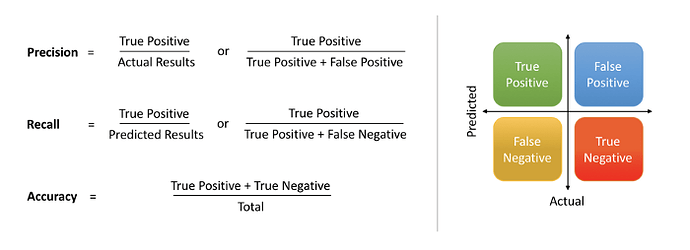# What is a recall?

What is a recall?• Precision : Precision is the ratio of correctly predicted positive observations of the total predicted positive observations.

Precision = TP/TP+FP

• Recall : Recall is the ratio of correctly predicted positive observations to all observations in the actual class

Recall = TP/TP+FN